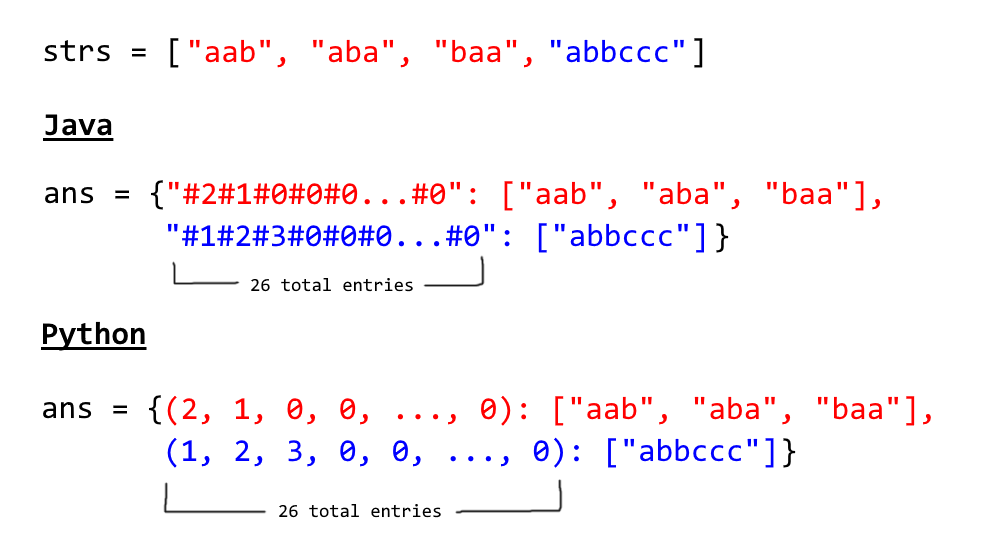#### Approach 1: Categorize by Sorted String

Intuition

Two strings are anagrams if and only if their sorted strings are equal.

Algorithm

Maintain a map ans : {String -> List} where each key is a sorted string, and each value is the list of strings from the initial input that when sorted, are equal to .

In Java, we will store the key as a string, eg. code. In Python, we will store the key as a hashable tuple, eg. ('c', 'o', 'd', 'e').Complexity Analysis

• Time Complexity: , where is the length of strs, and is the maximum length of a string in strs. The outer loop has complexity as we iterate through each string. Then, we sort each string in time.

• Space Complexity: , the total information content stored in ans.

#### Approach 2: Categorize by Count

Intuition

Two strings are anagrams if and only if their character counts (respective number of occurrences of each character) are the same.

Algorithm

We can transform each string into a character count, , consisting of 26 non-negative integers representing the number of 's, 's, 's, etc. We use these counts as the basis for our hash map.

In Java, the hashable representation of our count will be a string delimited with '#' characters. For example, abbccc will be #1#2#3#0#0#0...#0 where there are 26 entries total. In python, the representation will be a tuple of the counts. For example, abbccc will be (1, 2, 3, 0, 0, ..., 0), where again there are 26 entries total.Complexity Analysis

• Time Complexity: , where is the length of strs, and is the maximum length of a string in strs. Counting each string is linear in the size of the string, and we count every string.

• Space Complexity: , the total information content stored in ans.